## Introduction

The concern for dispersion stability is significant in colloidal dispersions. A colloidal dispersion consists of colloidal particles dispersed in a continuous fluid medium. Colloidal dispersions possess considerable application potential in various fields, including food, soil science, hydrology, catalysis, agrochemical, pharmaceutical, cosmetics, environmental science and technology, composite materials manufacturing and energy industries1,2,3,4,5,6,7,8.

In general, the attraction between colloidal particles leads to particle aggregation and phase separation of colloidal dispersions. Stability improvement can be achieved through either increasing repulsion or decreasing attraction between colloidal particles. Two traditional mechanisms for colloidal stability are electrostatic stabilization and steric stabilization. Electrostatic stabilization is the mechanism in which the attractive van der Waals forces are counterbalanced by the repulsive Coulomb forces acting between the charged colloidal particles. The steric stabilization of colloids involves additives that are added to the fluid medium to inhibit the coagulation of the particle suspension. The traditional methods for stability improvement include changing the property (such as pH value9) of the fluid medium or using additives. Additives usually include dispersants, such as surfactants and polymer9,10,11. Charged particles12,13,14,15,16 can also act as dispersants for colloidal stabilization in multi-component suspensions. Dispersants should be stably dispersed in the fluid medium to achieve good dispersion stability of colloidal particles. However, can the effect of mixing itself possibly contribute to the improvement of colloidal stability regardless of the stability of the dispersants?

For a colloidal dispersion of multi-component particles, the free energy change by particle mixing can be used to study colloidal stability. A system becomes more stable at lower free energy, G, which is defined as follows17: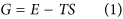where T, E and S are the temperature, energy and entropy of the system, respectively. According to Equation (1), free energy decreases with decreasing energy or increasing entropy. The free energy change after mixing multi-component particles, ΔGmix, can be calculated as follows: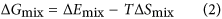where ΔEmix = Emix − Edemix, ΔSmix = Smix − Sdemix are the energy and entropy change of the system after mixing multi-component particles, respectively, the subscript mix and demix denotes the state after mixing and demixing (or before mixing), respectively. Since a negative change in free energy is thermodynamically favorable, if ΔGmix < 0, which means that mixing will decrease the free energy of the colloidal dispersion, mixing will be a spontaneous process and results in good dispersion of the multi-component particles. Otherwise if ΔGmix > 0, demixing is thermodynamically favored to decrease the free energy of the colloidal dispersion.

Although the entropically driven phase behaviours of binary colloidal dispersion systems have garnered considerable attention18,19,20,21,22,23,24,25,26, these works have focused on phase demixing26,27, and the function of entropy in the improvement of colloidal stability has been scarcely reported until now.

To evaluate the function of mixing entropy in colloidal stability, we mixed two types of particles and studied the phase behaviour of the binary suspension. To minimize the effects of electrostatic stabilization and steric stabilization, we mixed two types of unstably dispersed particles with electrostatic attractions. Moreover, to minimize other stabilization effects related to the difference in chemical composition, if any, we selected TiO2 spheres and platelets, which are particles with the same chemical composition but different shapes, as model particles for mixing.

## Results and Discussion

The average diameter of TiO2 spheres is approximately 20 ± 10 nm, and the TiO2 platelets are generally rectangular with the edge length in the range of 20–80 nm, as shown in Fig. 1a,b. The spheres and platelets were dispersed into deionized water having a pH value of 7.0. The zeta potentials of the sphere and platelet suspensions, which were measured by a zeta potential analyzer, were 5.73 and −4.11 mV, respectively. This finding indicates that the suspensions were unstably dispersed because of low absolute zeta potentials28; moreover, the electrical charges on spheres and platelets are positive and negative, respectively, thus the electrostatic forces between the spheres and platelets will be attractive if the spheres and the platelets are mixed.

A series of suspensions was prepared with the mass concentrations of spheres ranging from 0.002% to 0.50%, and platelets ranged from 0.002% to 0.24%. The binary suspensions were immobilized for observing the phase transition phenomenon after preparation. Figure 2 shows photographs of suspensions of 0.1 ± 0.001% spheres with platelets ranging from 0.002% to 0.24%. All samples were found to be fluid immediately after preparation. Two phase behaviours were observed at a minimum of 24 h after sample preparation. As shown in Fig. 2a, demixing with an interface observed in sample Nos 1–4 and Nos 11–13, whereas sample Nos 5–10 were stable and were well dispersed.

The optical absorbencies of the suspensions were measured by ultraviolet–visible spectroscopy to quantify dispersion stability. The absorbency variation of each sample was measured. Relative absorbency Rabs is defined as the ratio of absorbency A to the initial absorbency A0 at the middle of sample container after preparation: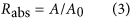Relative absorbencies of the samples were plotted in Fig. 2b. The dispersion stability of each sample was evaluated by the reciprocal of absolute value of slope of the curve in Fig. 2b, which is defined as the dispersion stability index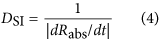The dispersion stability index of each sample is shown in Fig. 2c as a function of mass fraction of platelets. There are three zones, zone 1 for samples 1 to 4, zone 2 for samples 5 to 10, and zone 3 for samples 11 to 13. The results indicated that the addition of TiO2 platelets within the range of 0.008–0.12% improve the dispersion stability of 0.1% TiO2 sphere suspension (samples 5–10, zone 2), whereas an excessively small (samples 1–4, zone 1) or large number of platelets (samples 11–13, zone 3) do not induce improvement. In zone 1, samples 1 to 4 have similar dispersion stability because the amount of platelets addition is too small to have sufficient effect on the sphere suspensions. In zone 3, addition of platelets at relatively high concentration even decreased the dispersion stability of sphere suspensions due to depletion attraction between the nanoparticles, thus zone 3 is even lower than zone 1.

Two-phase behaviours were also observed at other concentrations of spheres ranging from 0.002% to 0.50%, and platelets ranging from 0.002% to 0.24%. A dynamic phase diagram (Fig. 3a) was constructed based on experimental observations. The salient feature of the phase diagram is the stable mixing region, in which spheres and platelets coexist stably. The stable/demixing division lines at concentrations of spheres and platelets that are lower than 0.002% are presented by dotted lines because of the lack of data given that experimental uncertainty would increase at such or lower concentrations. The results show that whether the binary suspension of spheres and platelets is stable depends on the mass concentrations of the spheres and platelets (ms and mp, respectively). The mixing of platelets with spheres improves the dispersion stability at proper concentrations.

At relatively high ms and mp, the binary suspensions were unstable (upper right region in Fig. 3a), and demixing occurred, which can be attributed to depletion attraction. Similar to the demixing mechanism for a colloidal rod–plate mixture26, the origin of demixing may be the excess excluded volume of platelet–sphere pair compared with platelet–platelet and sphere–sphere pairs. The TEM photographs of the supernatant and sedimentation of sample 12 are shown in Fig. 1c,d, respectively. The supernatant consists of both platelets and spheres (Fig. 1c), whereas sedimentation mainly consists of platelets (Fig. 1d). These findings confirm that the attractive depletion force induced by spheres result in the aggregation and sedimentation of platelets.

At relatively low ms and mp, the stability of the suspensions varies with the weight fraction, fp = mp/(mp + ms), of platelets in the sphere–platelet mixture. As shown in Fig. 3a, stable suspensions were observed at a medium fp in contrast with the demixing region, in which the particles tend to settle down at either relatively low fp or high fp.

The influence of mixing fraction of spheres and platelets on the dispersion stability of the suspensions is discussed as follows. According to Equation (2), free energy change decreases with decreasing energy change or increasing entropy change. For mixtures of spheres and platelets, the energy of mixing, ΔEmix, can be obtained similarly to the literature29 as follows: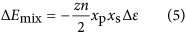where z is the coordination number; n = np + ns is the total number density of particles; and xp = np/n and xs = ns/n are the number fractions of platelets and spheres, respectively; the number density of the platelets and spheres are np = mpρcs/(ρvp) and ns = msρcs/(ρvs), respectively, where vp and vs denotes the average volume of a platelet and a sphere, respectively, ρ and ρcs is the density of the particles and colloidal suspension, respectively; Δε is the interaction energy between particles: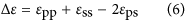where εpp, εss and εps refer to the interaction energy between the platelet–platelet, sphere–sphere and platelet–sphere pairs, respectively. The entropy change after mixing, ΔS, can be obtained by extending the Onsager theory similarly to the literature30,31 as follows: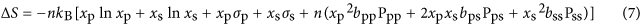where kB is the Boltzmann constant; σp and σs reflect the orientational entropies, which are functions of particle orientational distribution of the platelets and spheres, respectively. The variables bppPpp, bpsPps and bssPss represent the orientational distribution function dependent on excluded volumes of two platelets, a platelet and a sphere and two spheres, respectively. The entropy change in Equation (7) consists of three terms related to mixing, orientation and excluded volumes.

The interaction energy between particles as shown in Equation (6) is difficult to determine; meanwhile, the balance among the three entropy terms in Equation (7) is subtle, and calculation in theory or by simulation presents a formidable challenge30. However, the mixing entropy ΔSmix, that is, the change in entropy related to mixing, can be simply obtained17,30,31 as follows: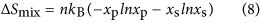The dimensionless mixing entropy,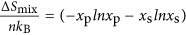, is plotted according to Equation (8) and shown in Fig. 3b. The result indicates that mixing particles increases the entropy of the suspension. The dimensionless mixing entropy first increases and then decreases as xp increases, reaching the maximum at xp = 0.5. According to Equation (1), free energy decreases with increased entropy. Given that a system becomes more stable at lower free energy, the mixing entropy favours the improvement in dispersion stability of colloidal suspensions.

Considering that the dimensionless mixing entropy reaches its maximum at xp = 0.5 for given number density of particles n, we plotted a phase diagram versus the number density of the platelets and spheres, as shown in Fig. 3c, with the line xp = 0.5 present. For comparison, the line fp = 0.5 (corresponding to mp = ms) is also shown in Fig. 3a. Interestingly, the line fp = 0.5 does not divide the stable region into two equal parts, with smaller stable region above the line compared with the larger region below the line. By contrast, the line xp = 0.5 divides the stable region into two parts of similar size. The coincidence implies that mixing entropy may perform a dominant function in the dispersion stability of binary suspensions. At relatively low xp or high xp (corresponding to relatively low fp or high fp), the mixing entropy is low (Fig. 3b) and tend to result in positive free energy change for given temperature and energy change according to Equation (2). Since positive free energy change due to mixing is unfavourable for stable dispersion of a colloidal system, the spheres and platelets tend to separate, then aggregate and settle down, in accordance with the phase diagram as shown in Fig. 3a,c.

With increasing concentration, the effect of interaction energy on the stability of colloidal suspension is less effective compared to mixing entropy, that’s why the stable/demixing division lines do not parallel the xp = 0.5 or fp = 0.5 line (Fig. 3a,c). Interaction energy of particles before and after mixing both increase with increased concentration, but mixing would not change the energy density because the temperature of the suspension is unchanged for the following reason. The concentration of particles in the colloidal suspensions is normally very low. There are many molecules from the continuous phase (in our case is water). So the temperature is determined by the continuous phase which was maintained at constant room temperature. In contrast, mixing increases entropy, and higher mixing entropy ΔSmix can be obtained by increasing either number density of particles (n) or (−xplnxp − xslnxs) according to Equation (8). Thus at higher n, a lower (−xplnxp − xslnxs) is enough to obtain the required mixing entropy ΔSmix for a negative free energy change (corresponding to stable mixed suspension) according to Equation (2). The (−xplnxp − xslnxs), which is the dimensionless mixing entropy (ΔSmix n−1 kB−1) according to Equation (8), is lower at relatively higher or lower number fraction of platelets (or spheres) as shown in Fig. 3b. Therefore, with increased nanoparticle concentration (corresponding to increased n), the suspensions can be stable even at relatively higher or lower number fraction of platelets (or spheres), resulting in wider stable region between the stable/demixing division lines (Fig. 3a,c).

To quantitatively understand the boundaries between stable suspension and demixing regions as shown in Fig. 3, one would have to calculate also the particle interaction term ΔEmix in Equation (2) or Equation (5), which is indeed difficult as we mentioned above. It would be the challenge of future theoretical study.

Here, we shall just qualitatively discuss the collaborative effects of both particle mixing and particle interaction on the colloidal stability of binary suspensions. A 3D schematic phase diagram is proposed in Fig. 4 to illustrate both the effects. It is a combination of phase diagrams of suspensions reported in the literature and our work here. The solid body in the 3D phase diagram represents the stable region. Outside surfaces of the 3D phase diagram are also presented for studying the effects of particle mixing or particle interaction. A limiting case for the binary suspension is a pure sphere suspension without depletion agent. The pure sphere suspension is stable at dilute concentrations while aggregation occurs above certain concentration, mc, which depends on the strength U of the interaction. A power law function was used indicating the boundary of mc on U32. This phase transition is shown in the front surface of the phase diagram, which is consistent with the literature32. For binary suspensions, the stable region decreases with the increase in particle attraction, as shown in the 3D phase diagram. When the particle interaction is 0, the binary suspension is stable at low sphere and platelet concentrations; conversely, the suspension becomes unstable and demixing occurs at high concentration because of the depletion attraction of platelets and spheres. This situation is shown in the bottom surface of the phase diagram, which is consistent with the hard particle model with no attractive interactions32. However, with the particle interaction increase, particle aggregation would become significant. At high sphere or platelet concentrations, the stable region decreases remarkably because of both particle interaction and depletion, and a typical case is shown in the back surface. If particle interaction is high enough, the stable region decreases to the case as shown in the top surface, which is in accordance with the phase diagram as shown in Fig. 3 based on our experimental data. The findings suggest that both effects of particle mixing and particle interaction should be considered in the study of colloidal stability of binary suspensions.